# Inter Maths Solutions for The Straight Line Exercise 3(d) Solutions

The Straight Line Exercise 3(d) Maths 1b textbook Chapter 3 Inter Solutions are given.

Study the textbook lesson The Straight Line very well.

All problems with answers are given. These are very simple to understand.

Observe the example problems and solutions in the textbook. Try them well.

Observe the given solutions and try them in your own method.

You can also see

Inter Maths 1A textbook solutions

Inter Maths 1B textbook solutions

Inter Maths IIA textbook solutions

Inter Maths IIB textbook solutions

The Straight Line

Exercise 3(a)

Exercise 3(b)

Exercise 3(c)

Exercise 3(d)

Exercise 3(e)

M

# Chapter 3 The Straight Line Solutions

Chapter 3 The Straight Line

Exercise 3(d)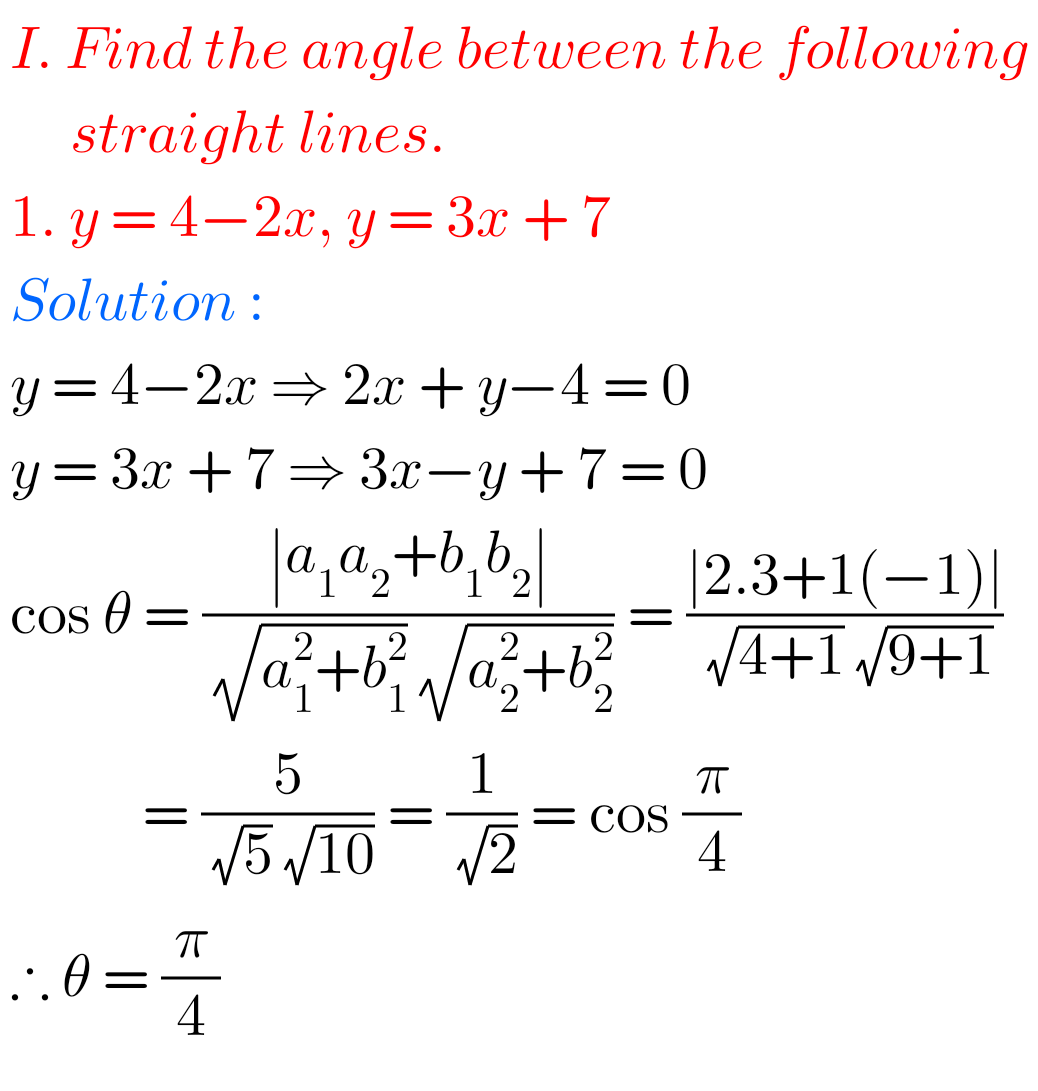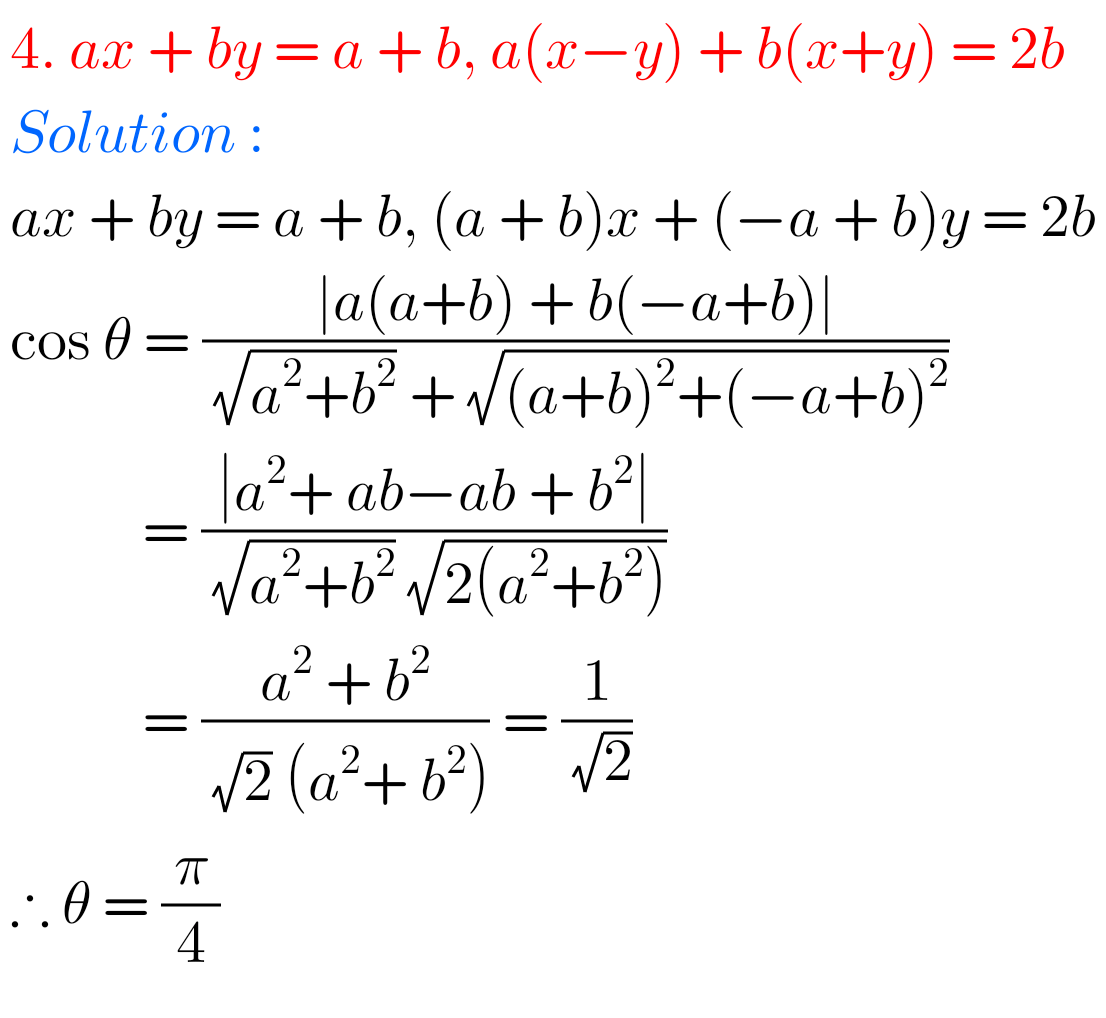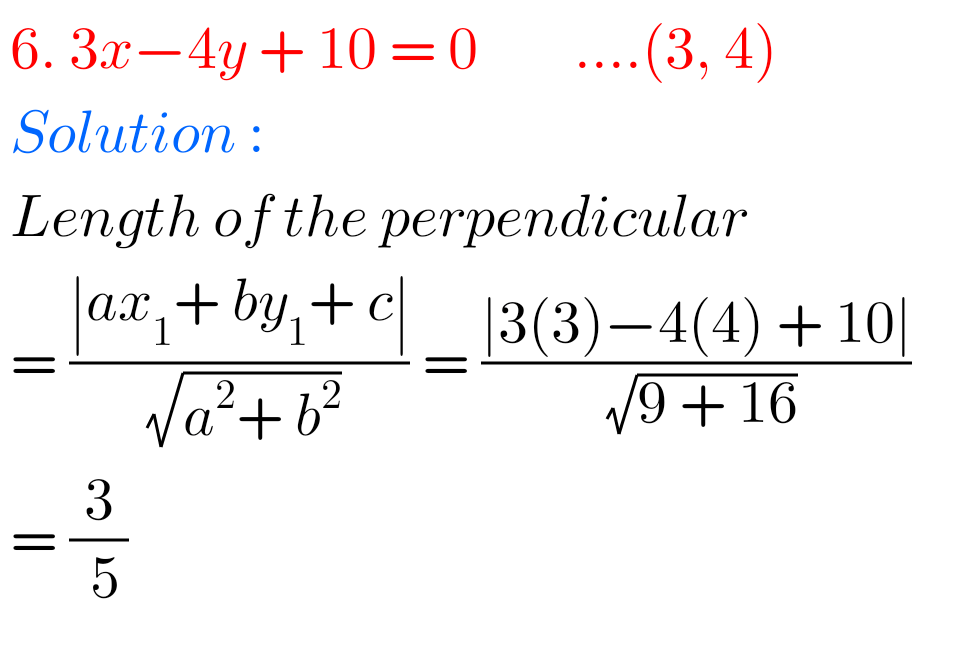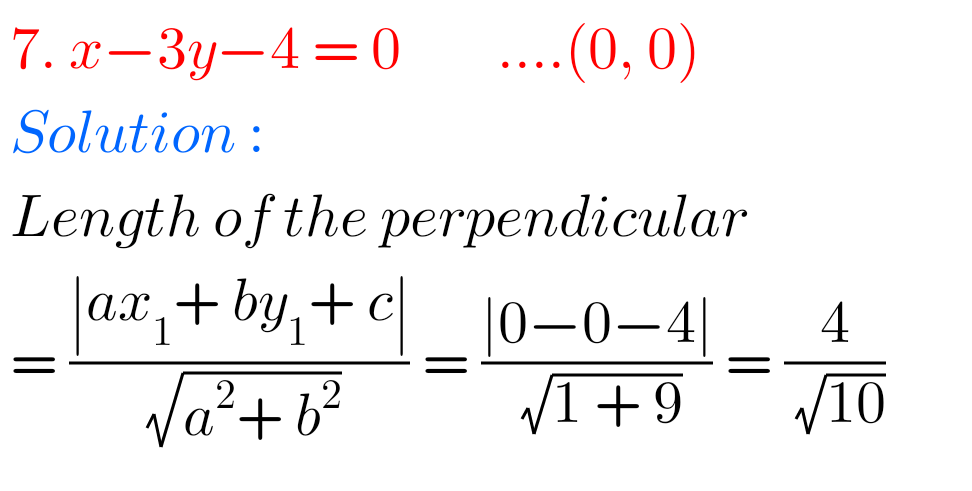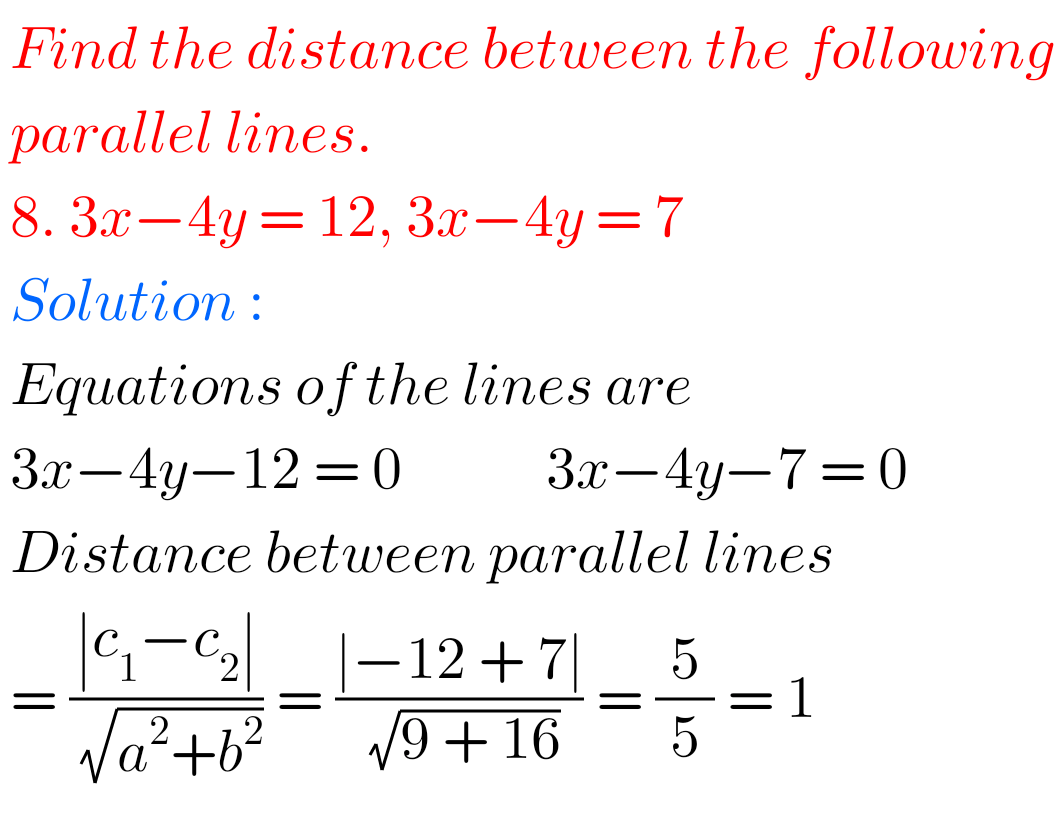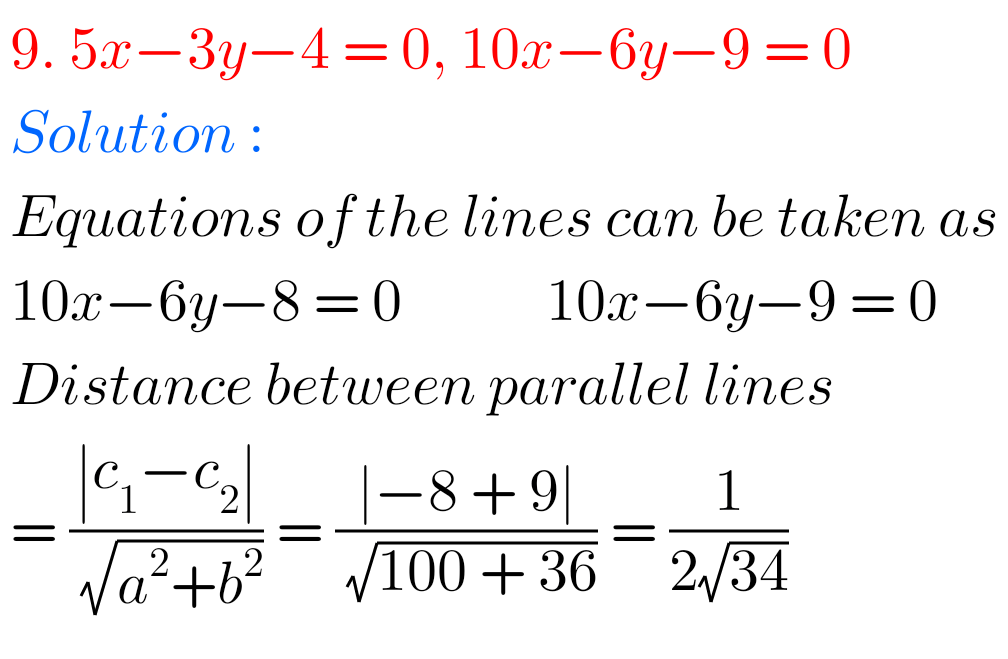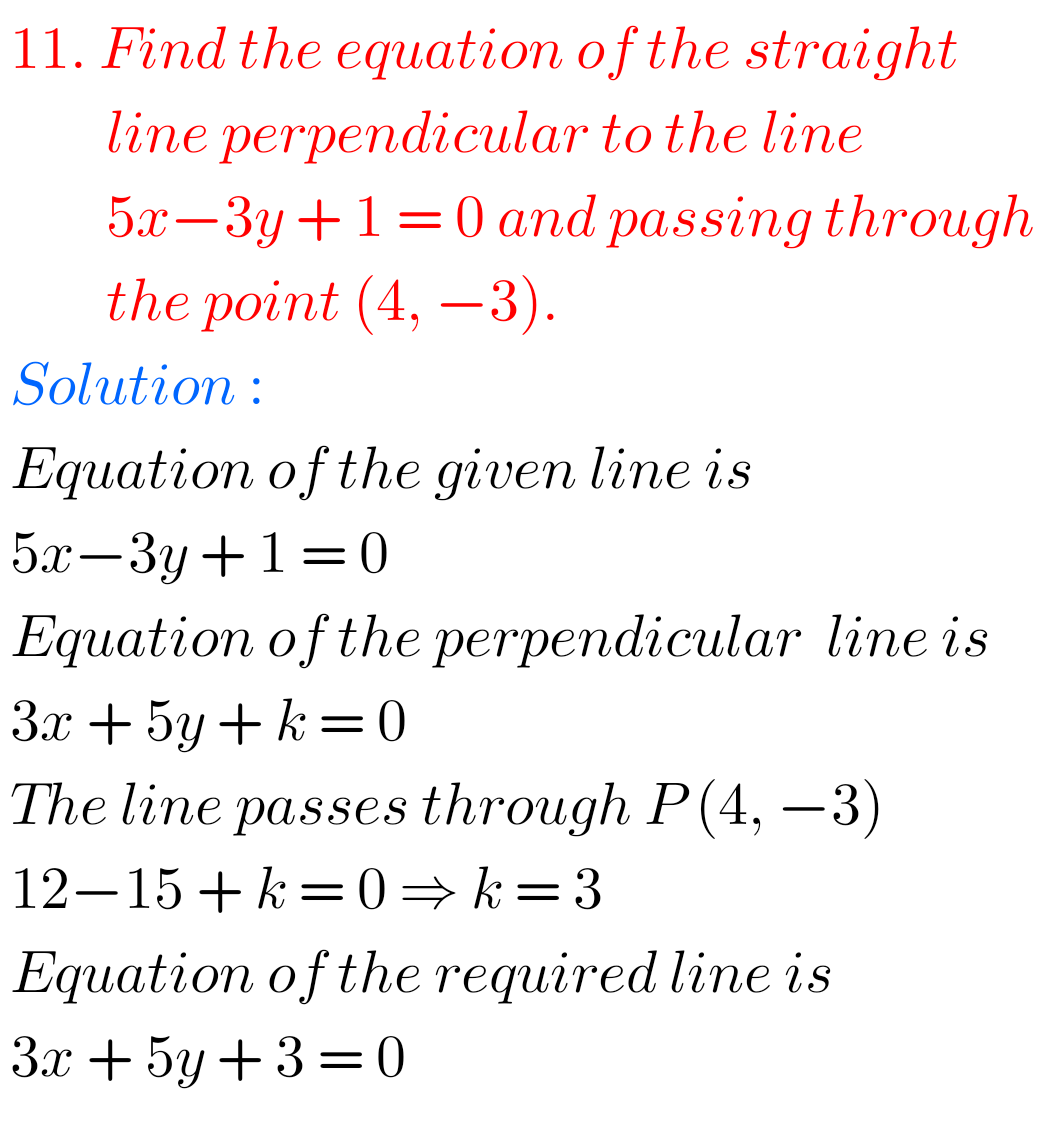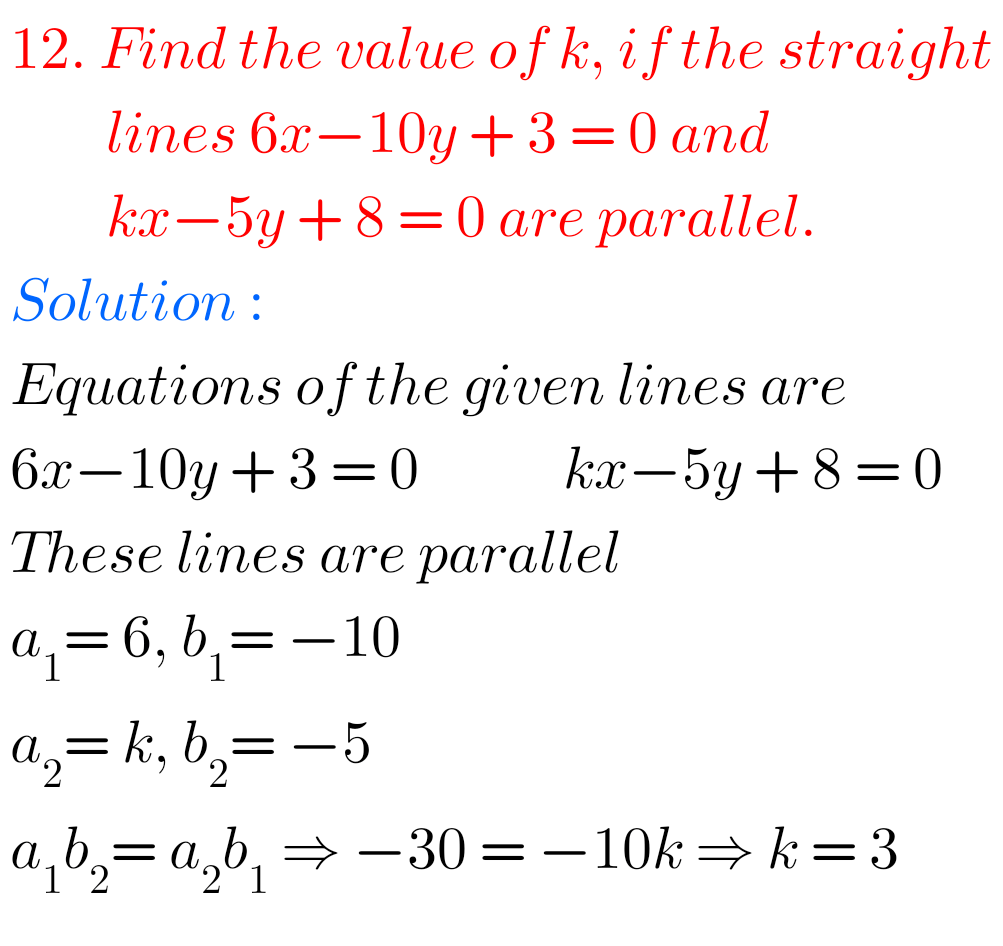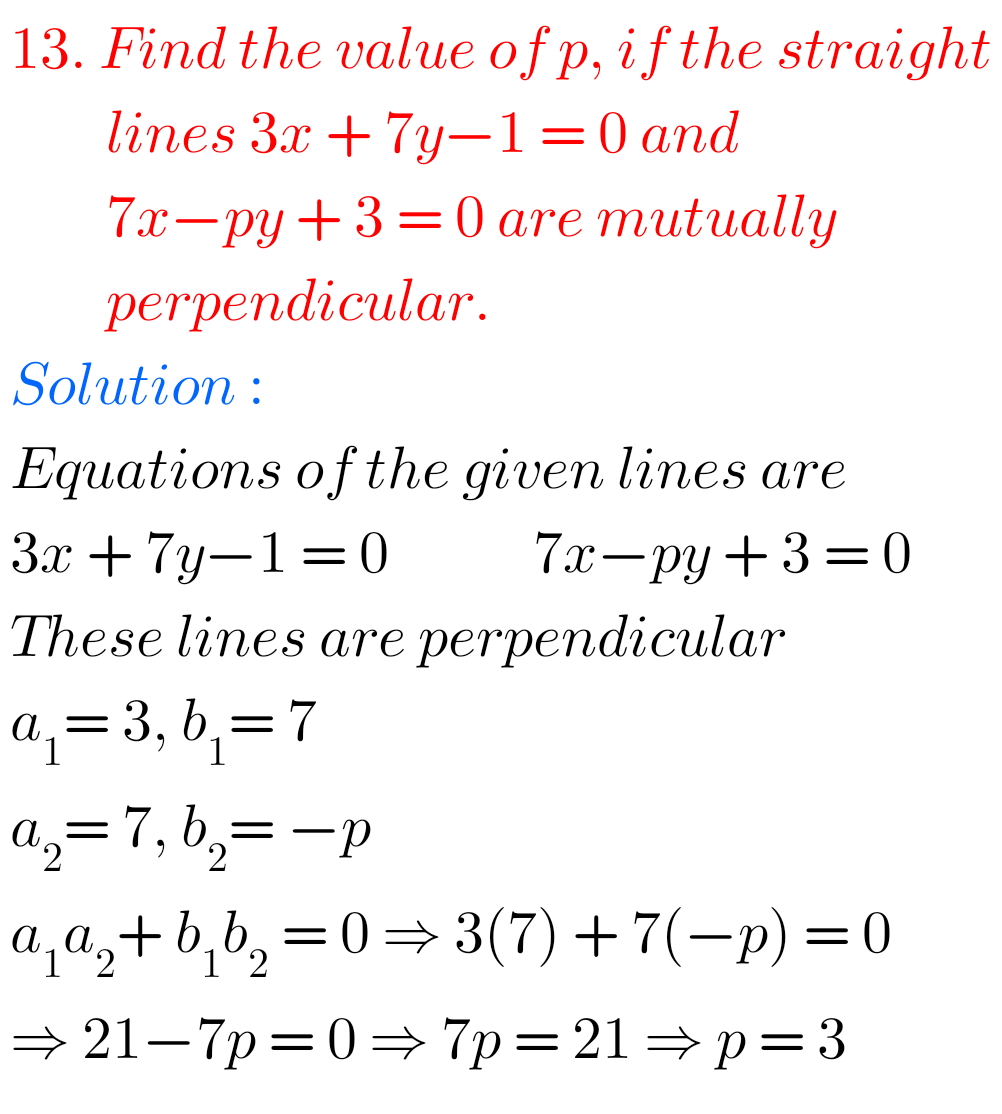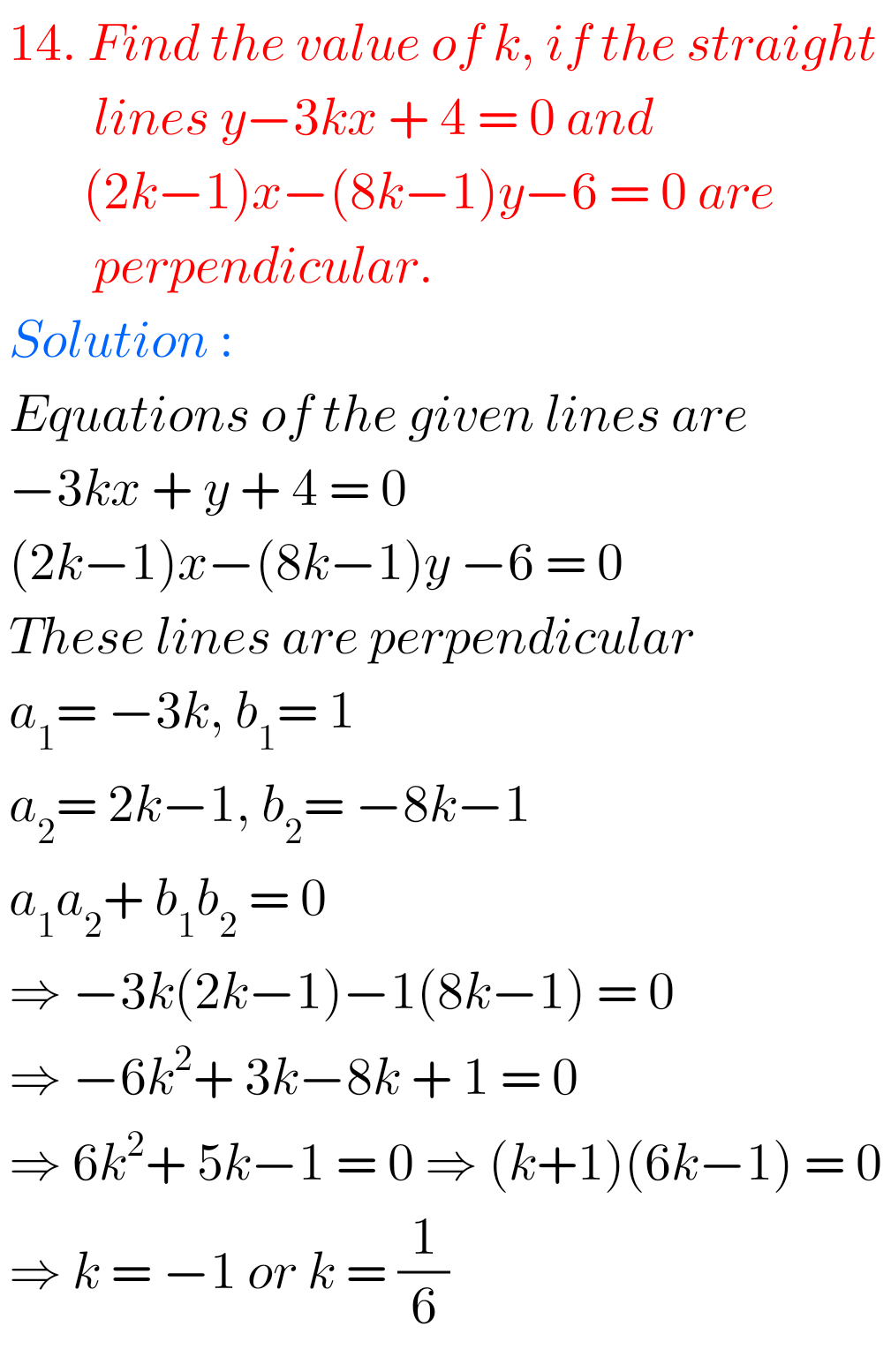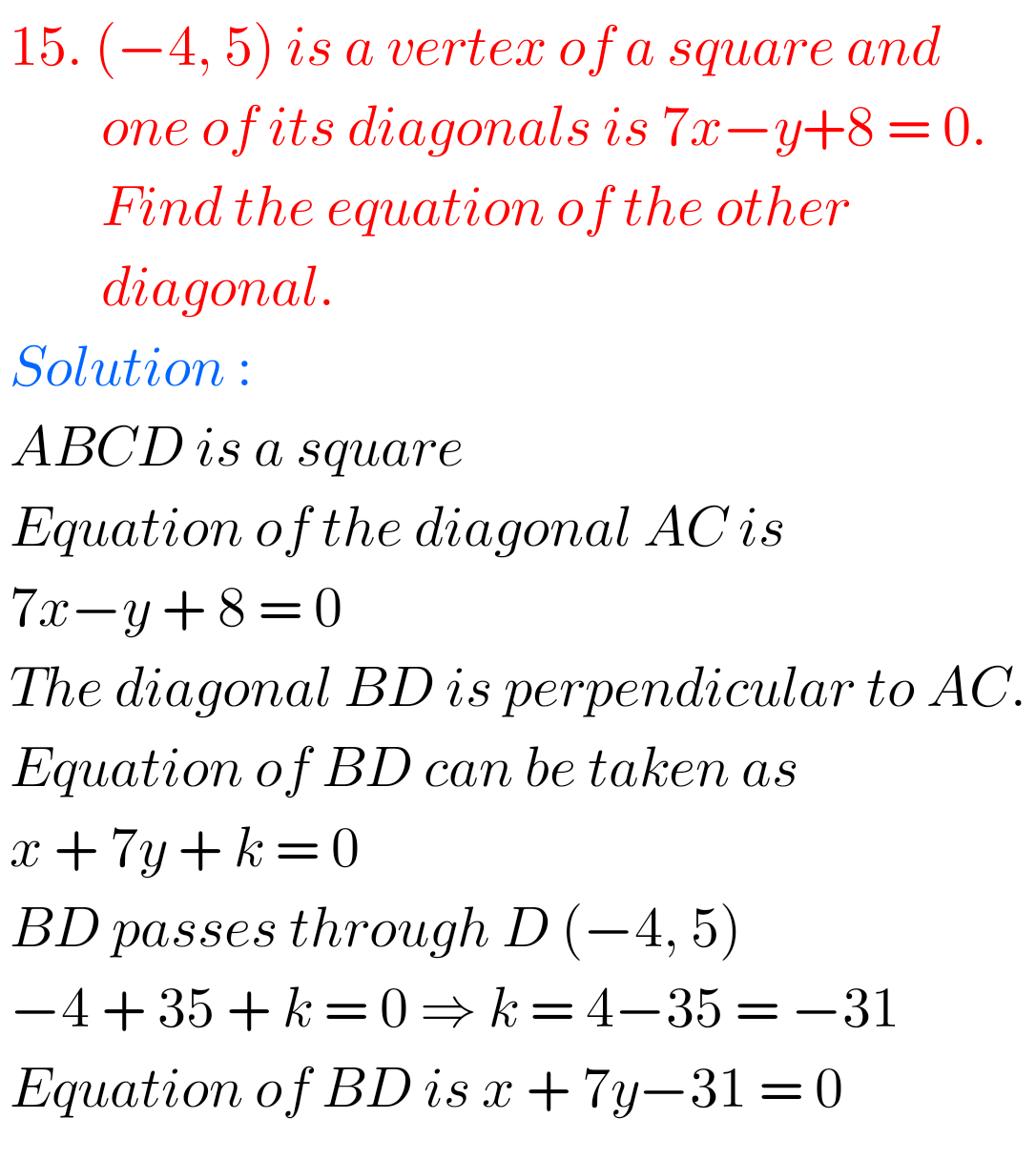M

## Maths Solutions for exercise 3(d) The Straight Line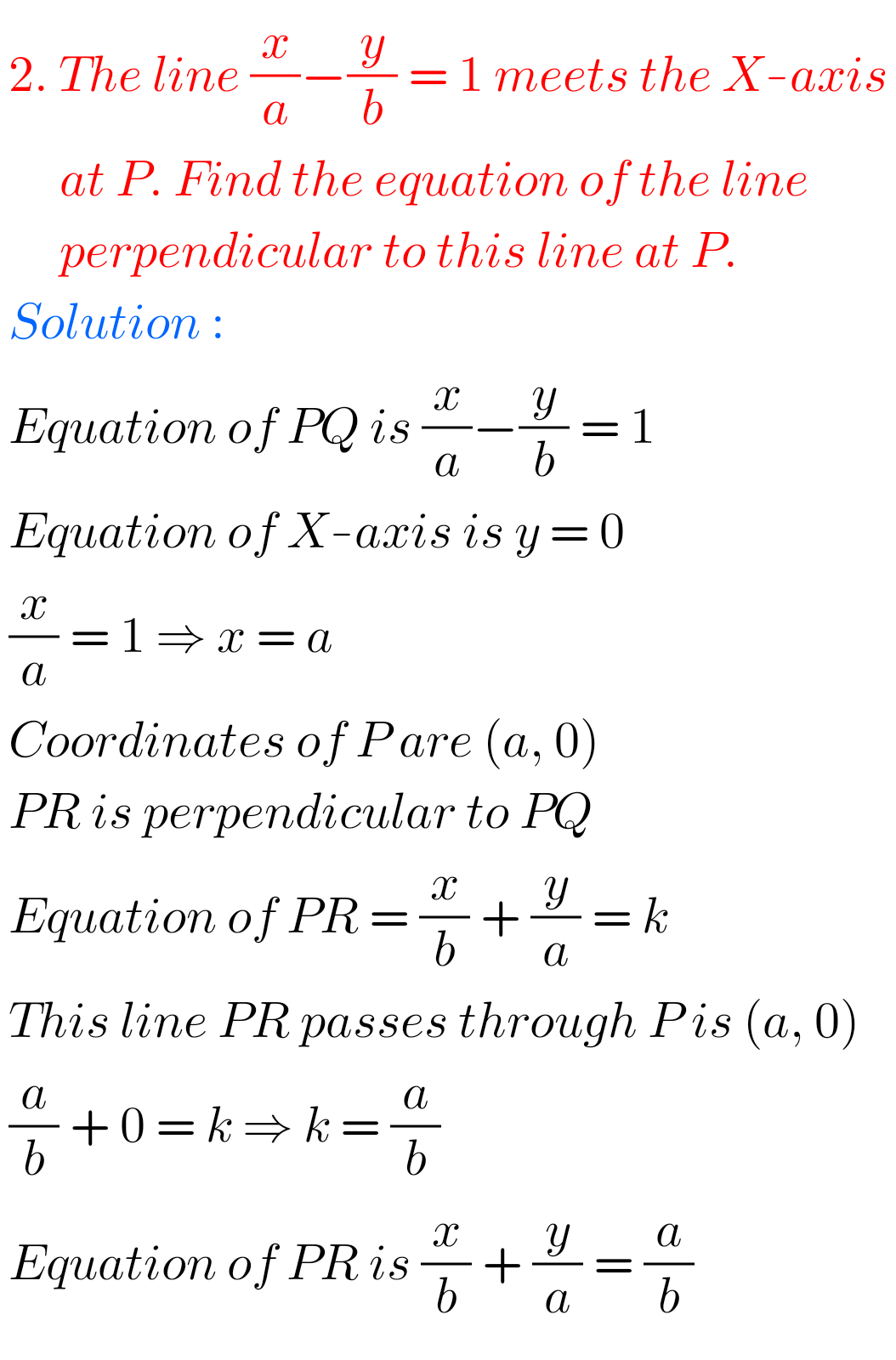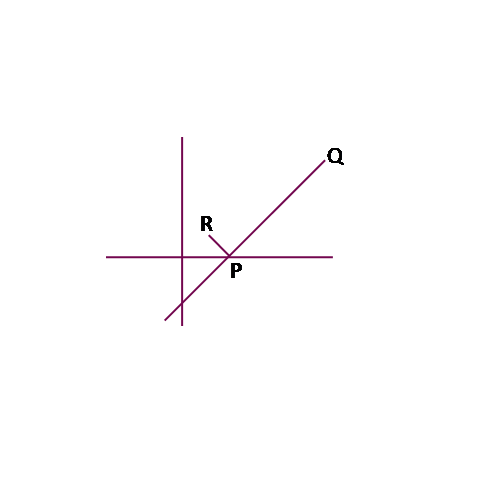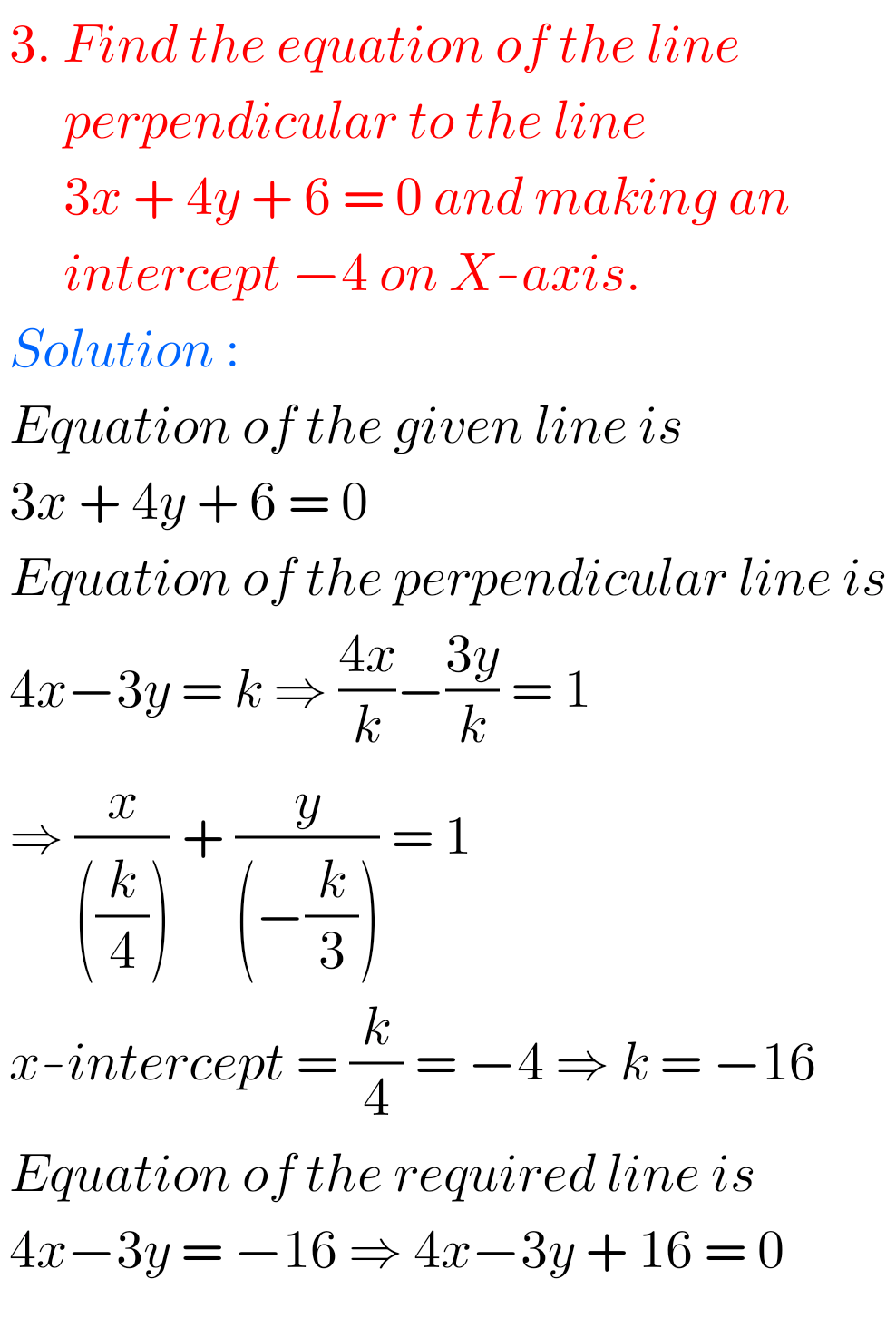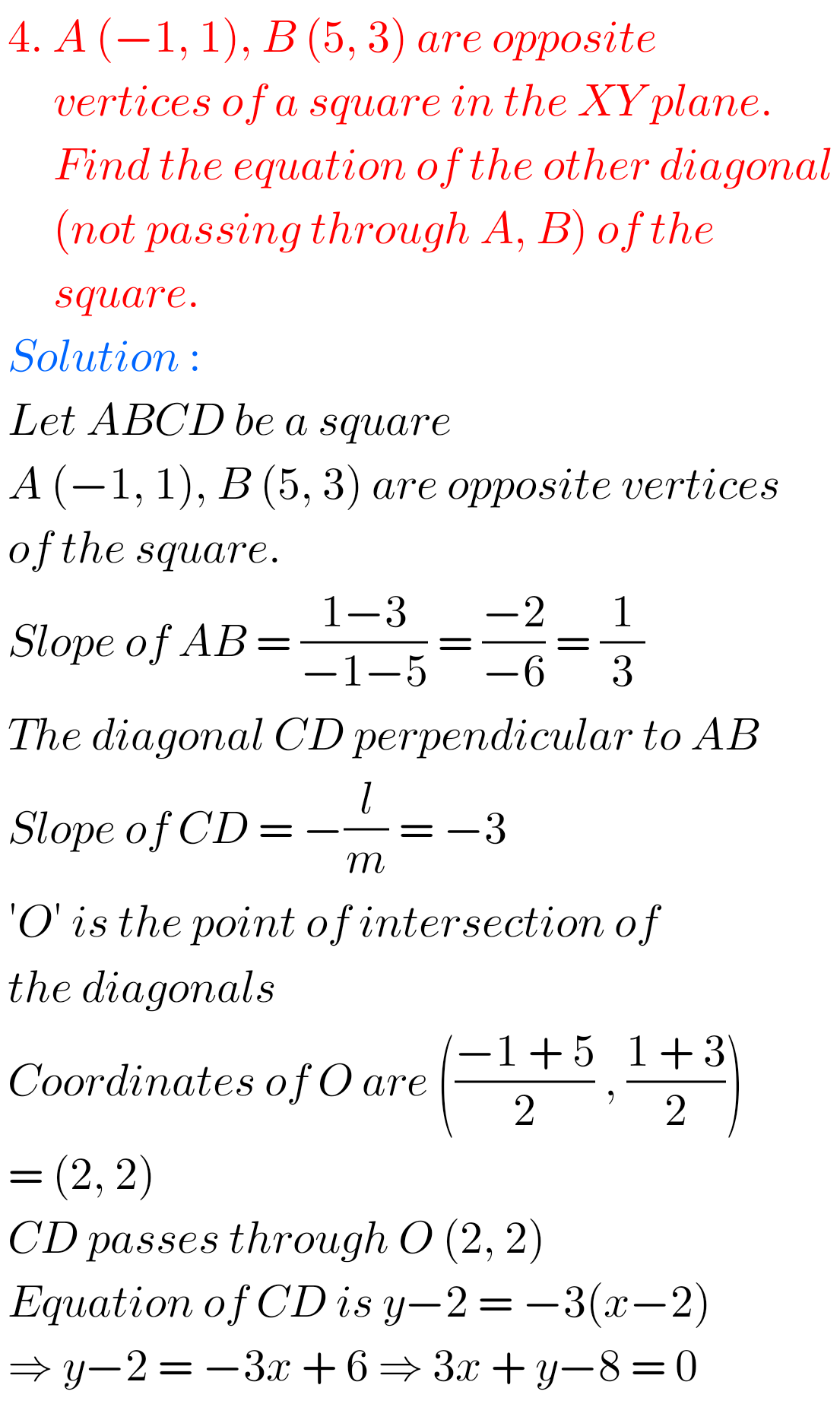7. Q

Figure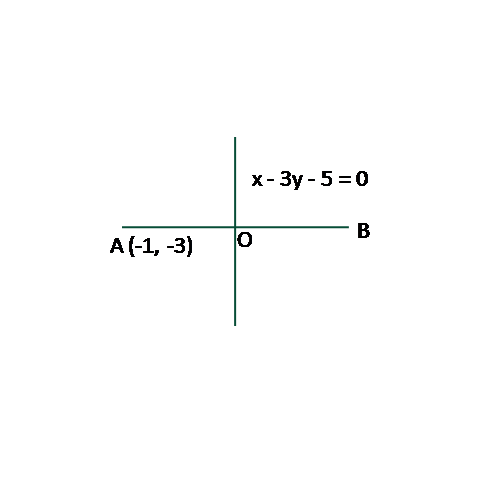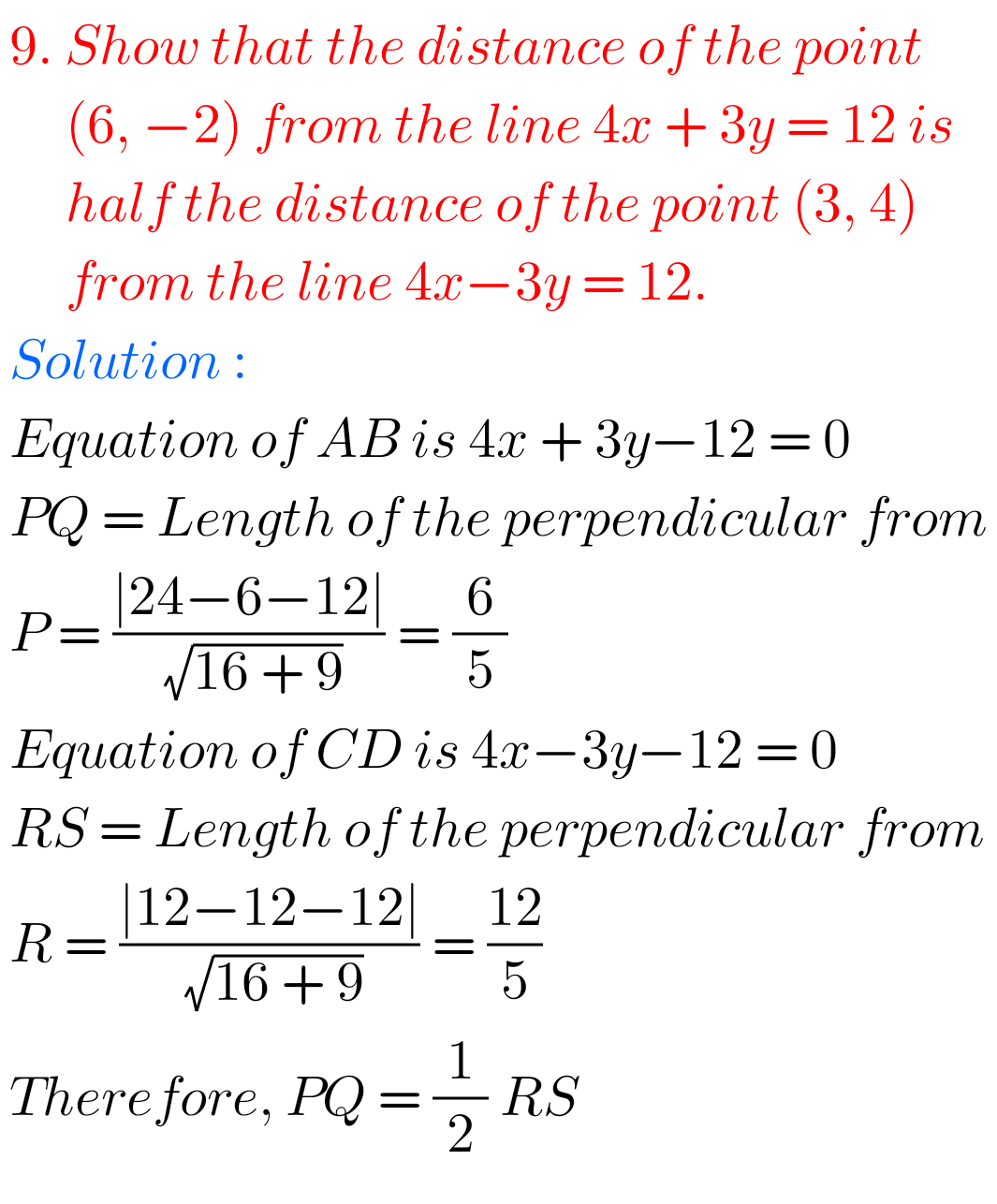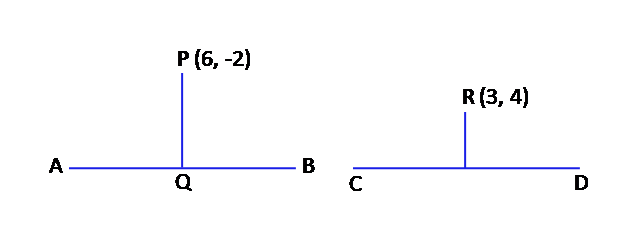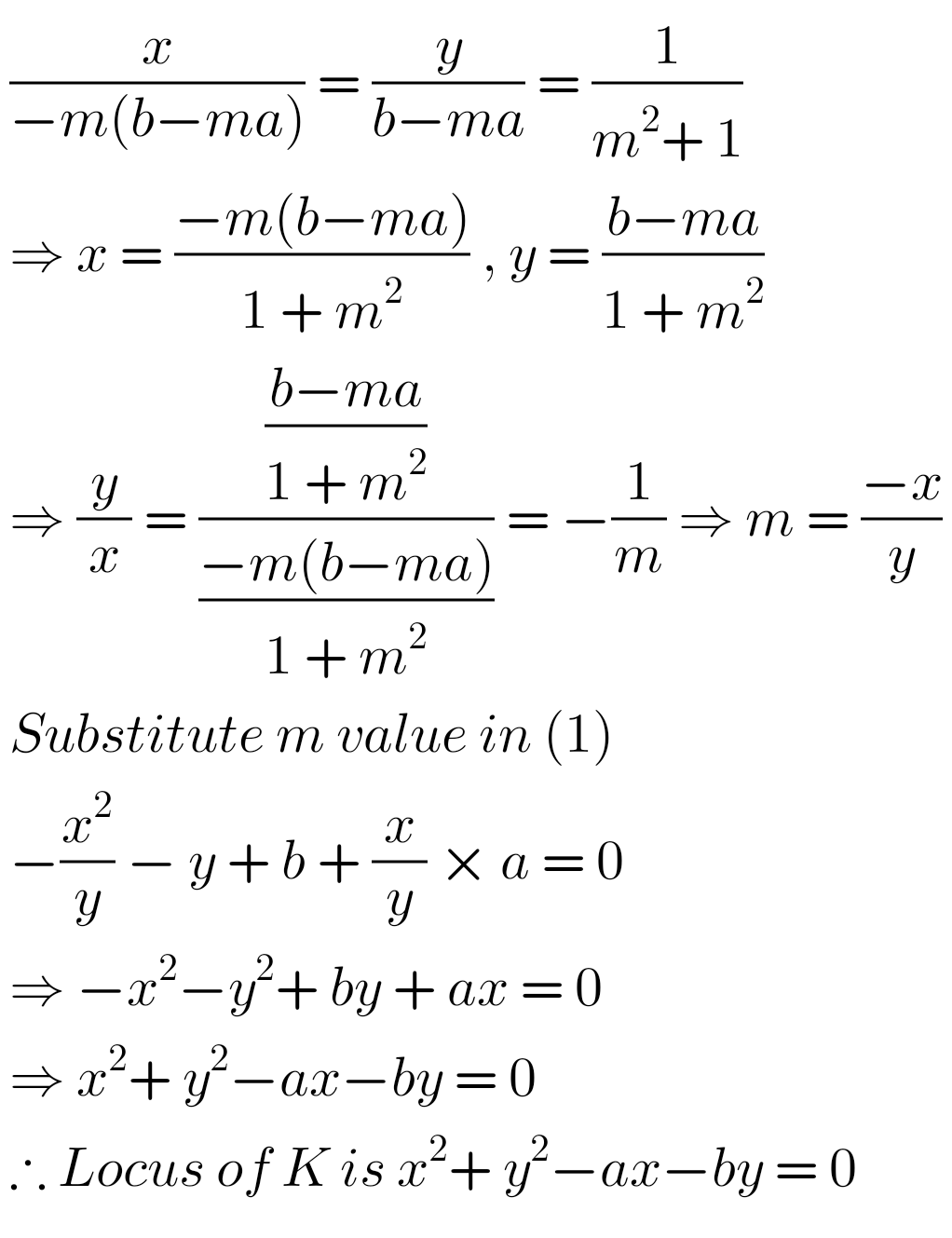## Exercise 3(d) Solutions The Straight Line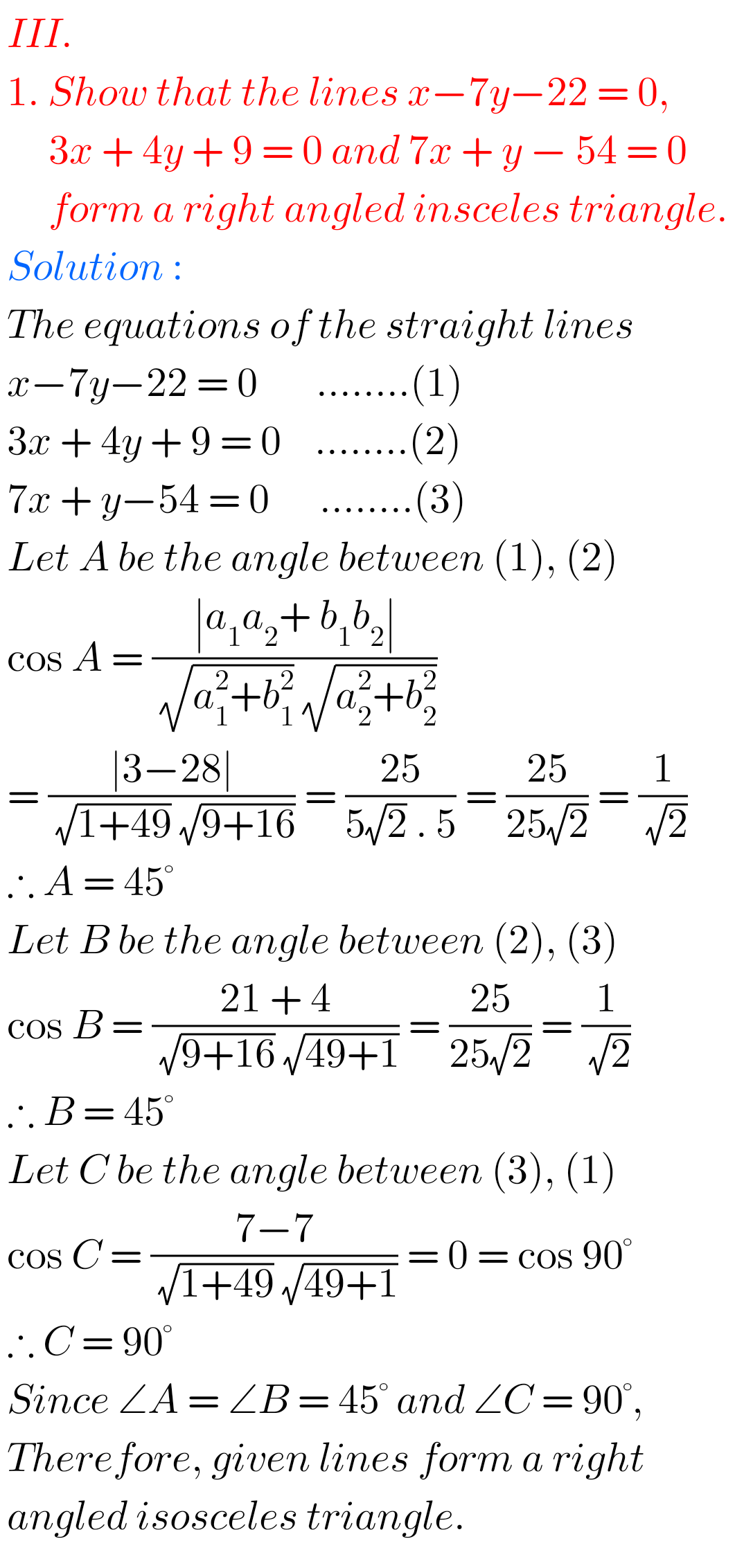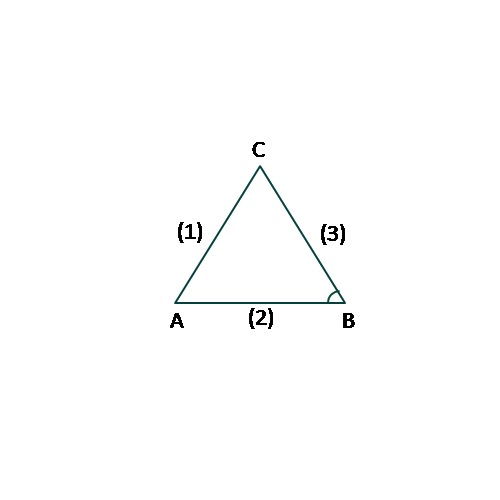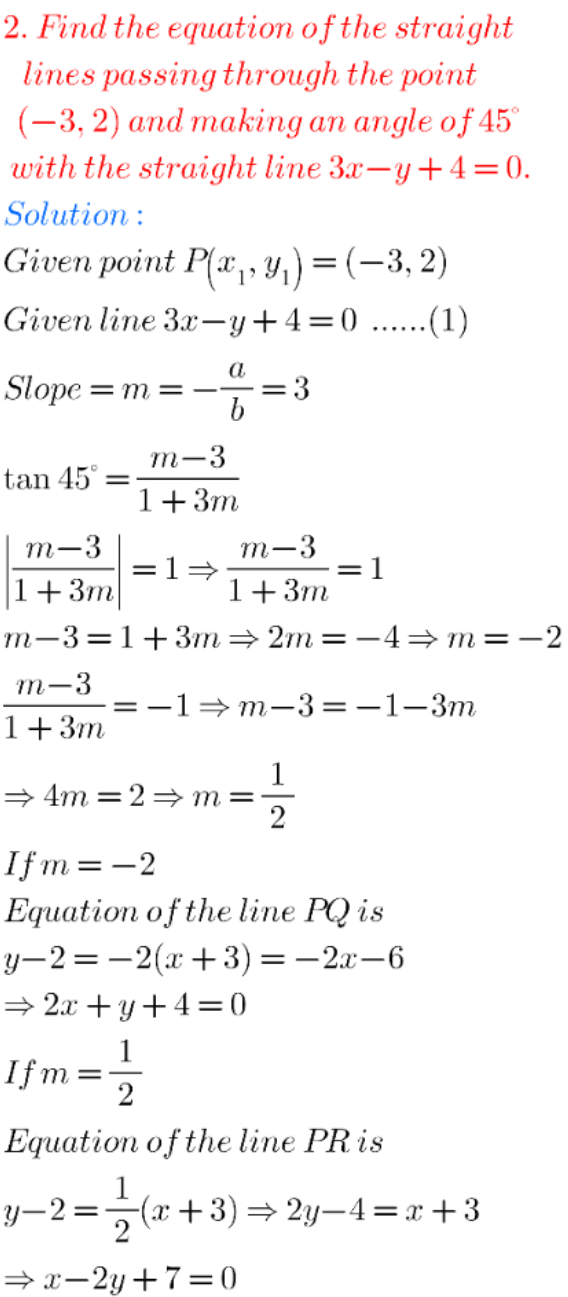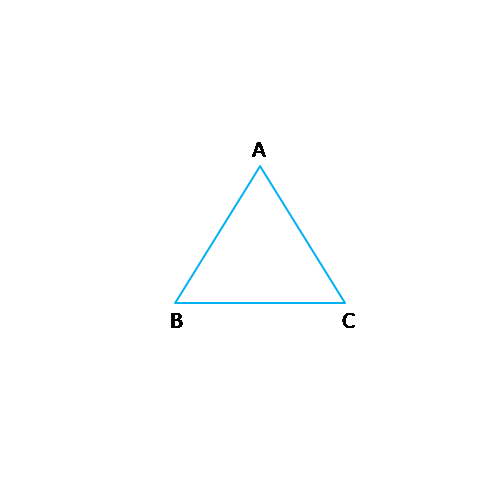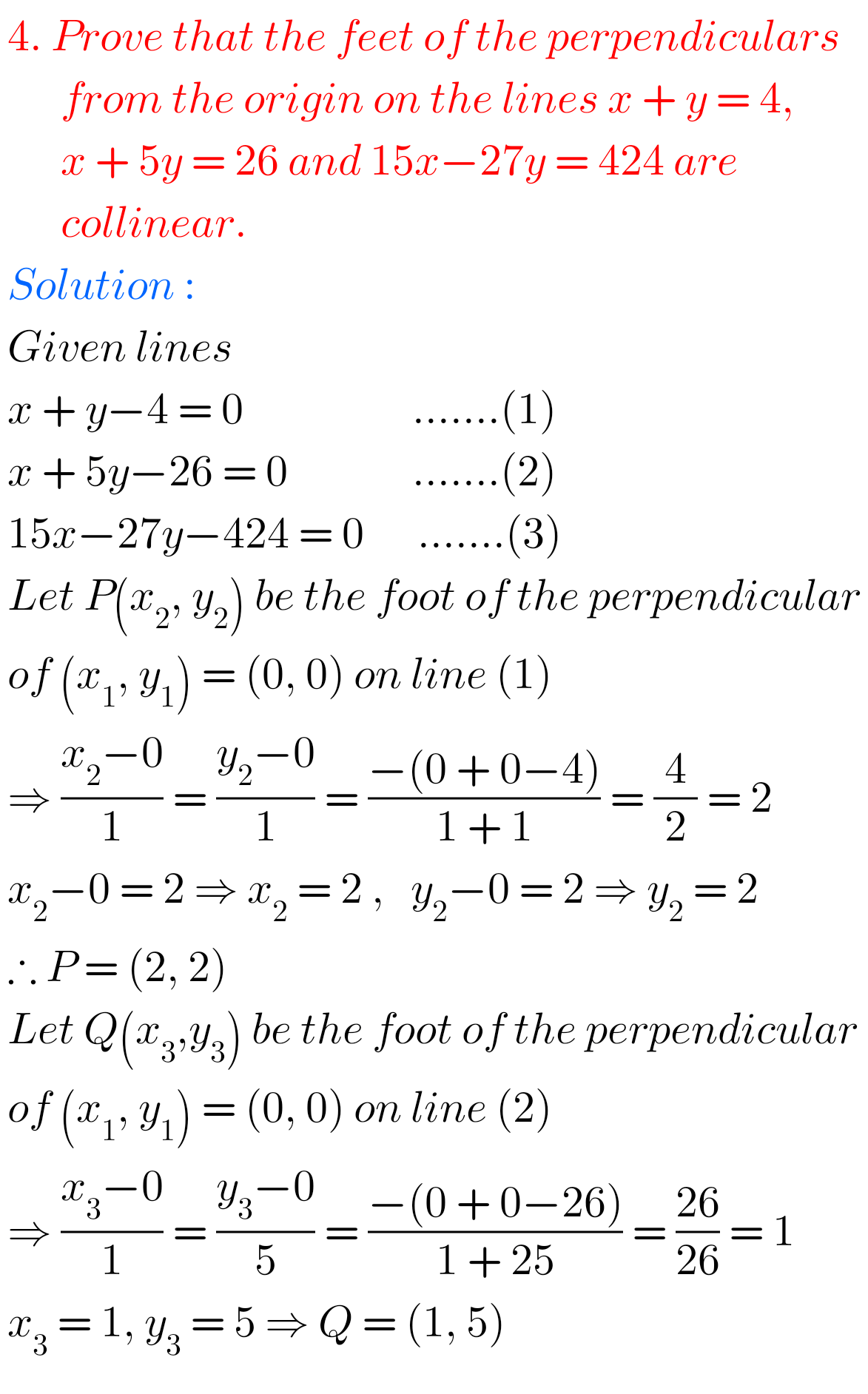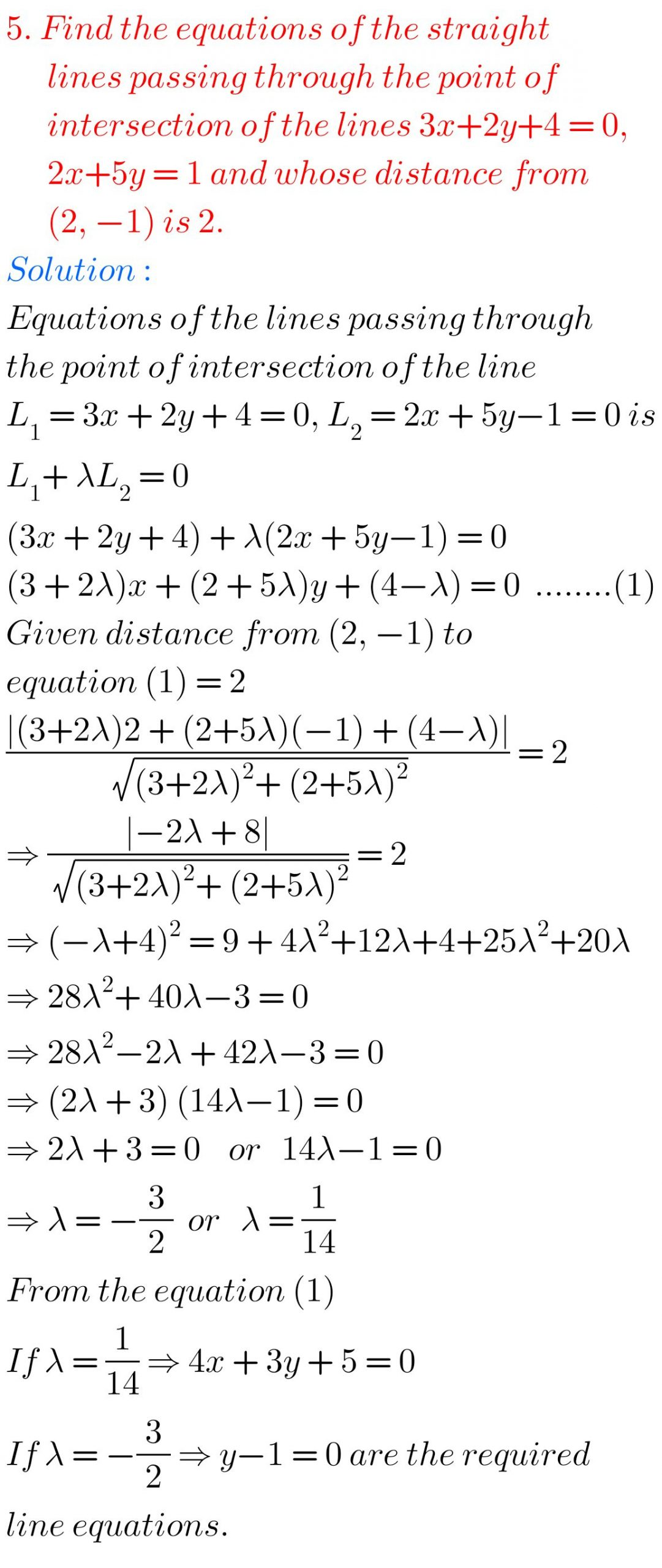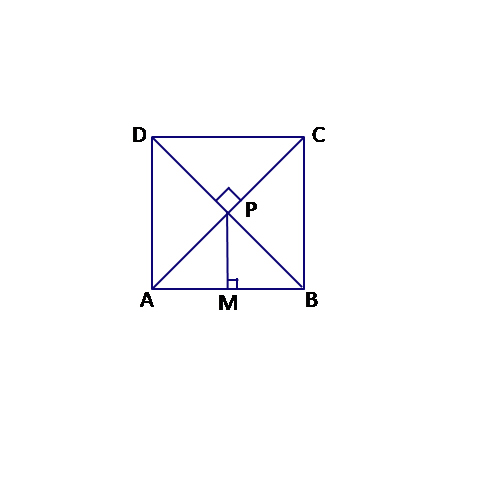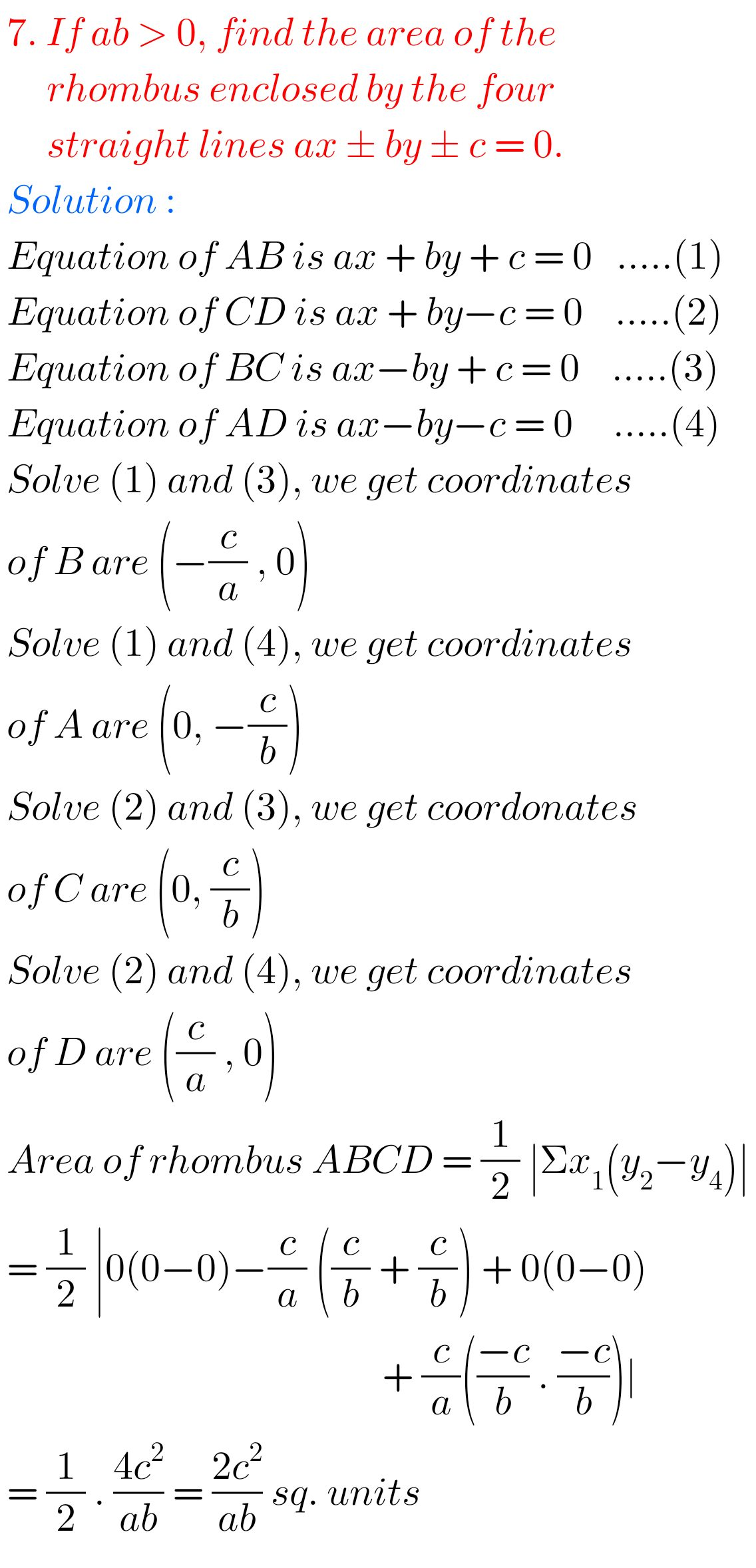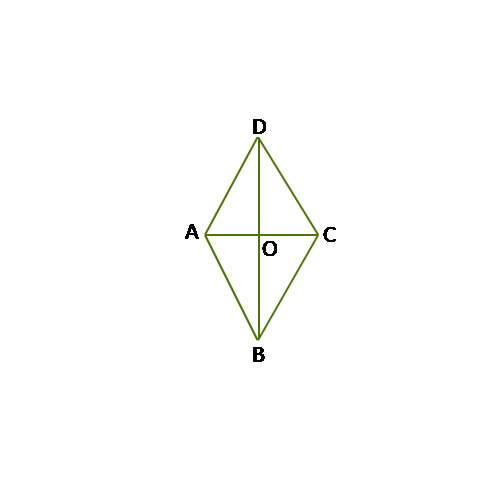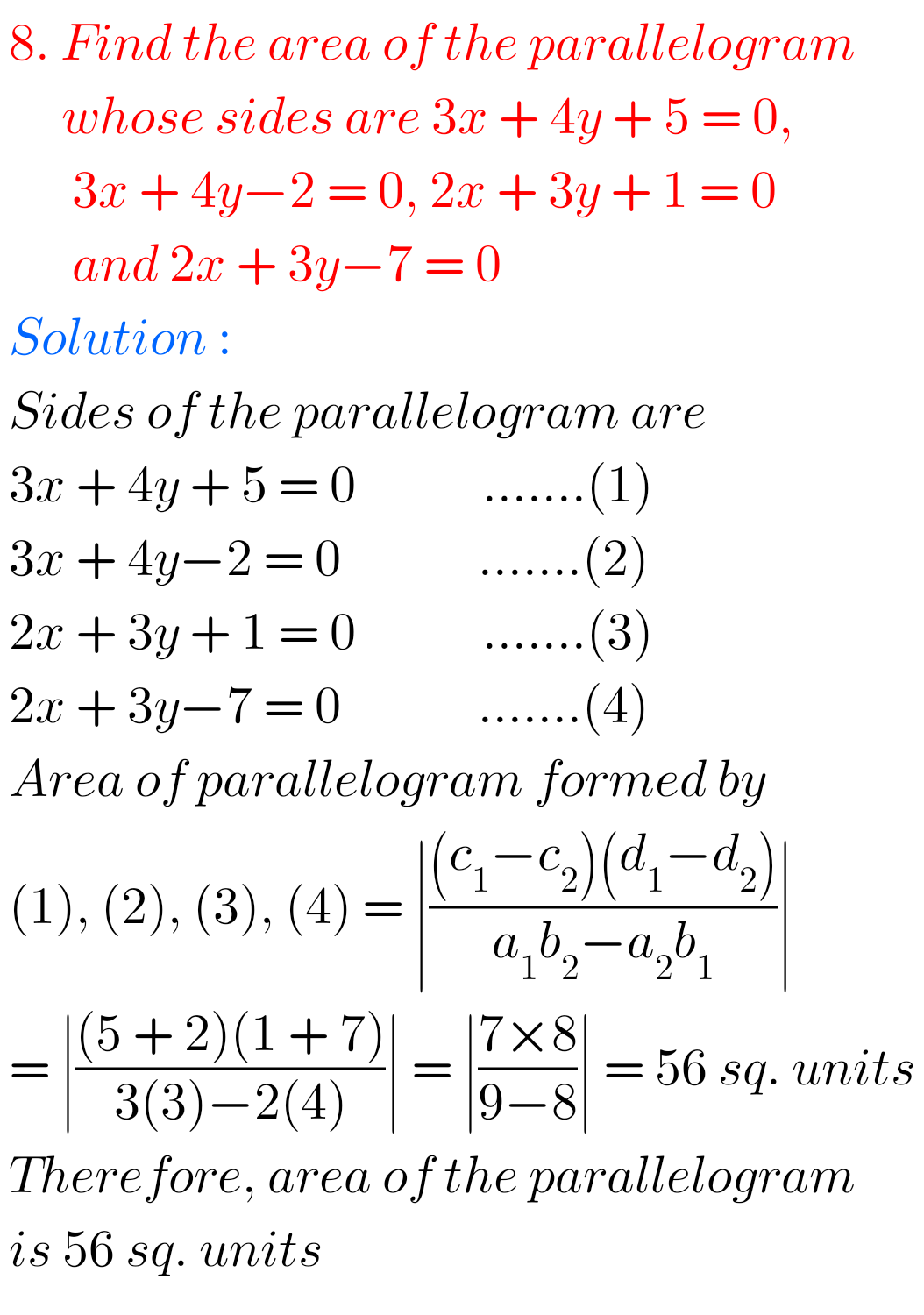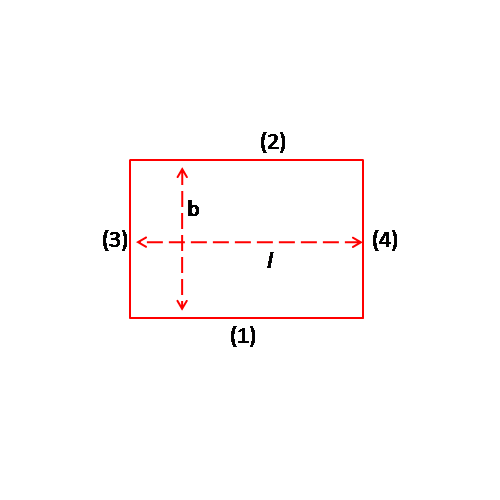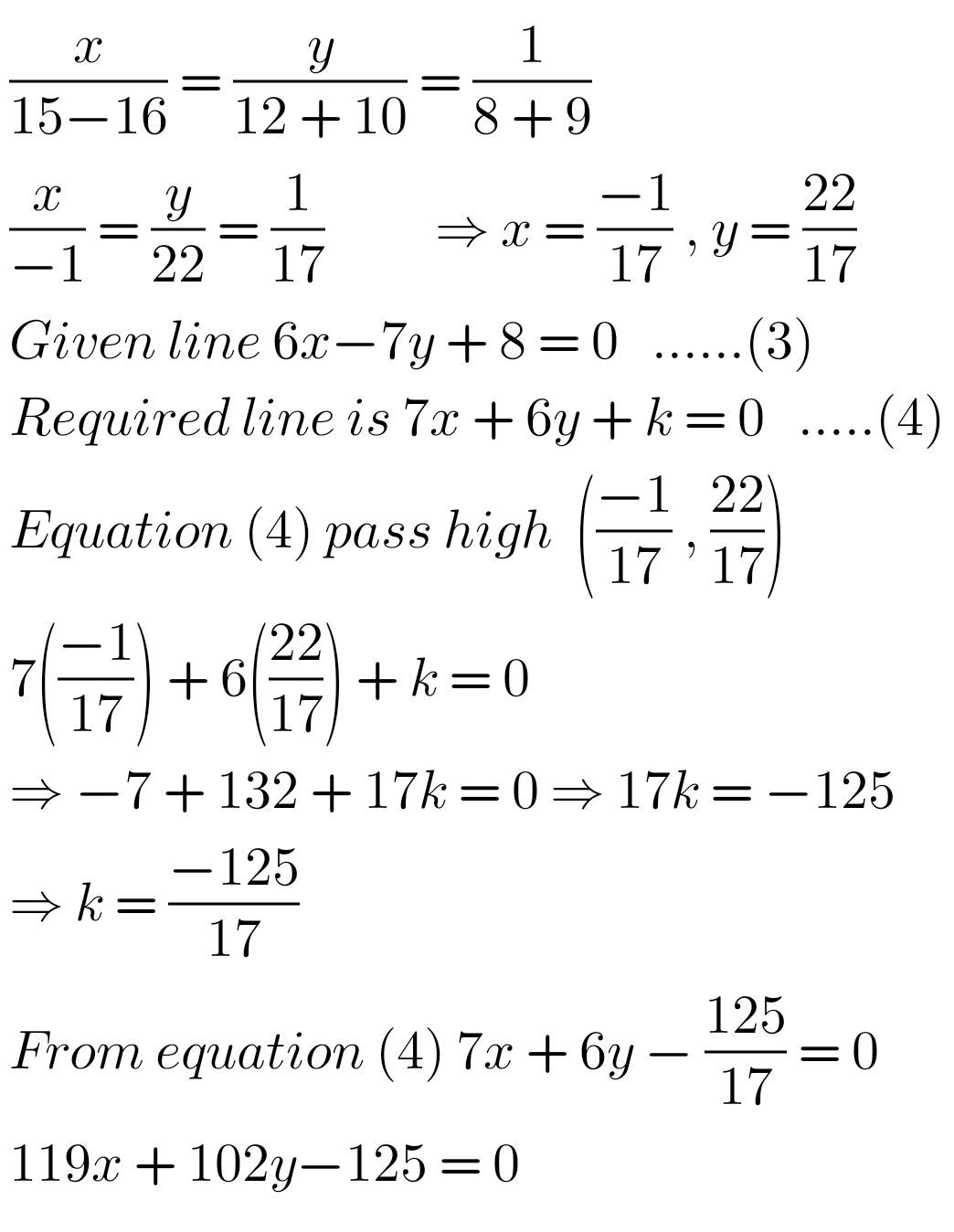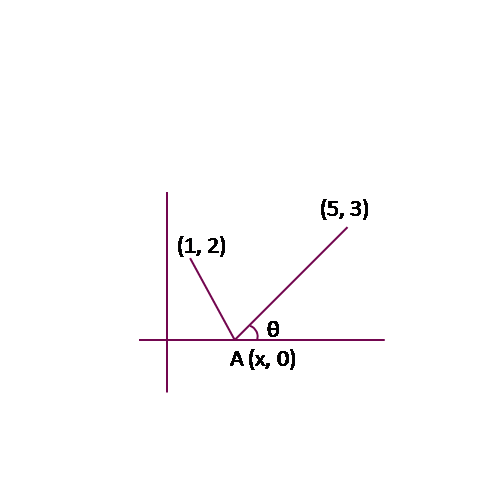Note : Observe the solutions and try them in your own method.